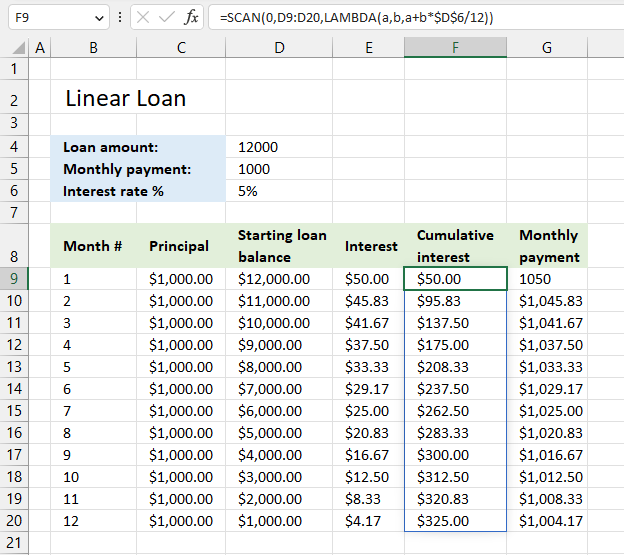Author: Oscar Cronquist Article last updated on March 13, 2023The SCAN function passes all values in an array to a LAMBDA function, the LAMBDA function calculates new values based on a formula you specify. It then returns an array with the same size as the original array. (This is not always the case.)

## 1. SCAN Function Syntax

SCAN([initial_value], array, lambda(accumulator, value, calculation))

## 2. SCAN Function Arguments

 Argument Description [initial_value] Optional. Lets you specify a starting value. array Required. A cell reference to a cell range or an array. lambda( accumulator, value, calculation) Required. A lambda function with three arguments: - accumulator - value - calculation accumulator Required. This is the sum the SCAN function returns. value Required. Iterates through each value in the array or cell range. calculation Required. A calculation containing the accumulator and value parameters.

## 3. SCAN Function ExampleThis example demonstrates a formula that calculates cumulative interest based on a cell range containing loan balance numbers (D9:D20).

The table in the image above shows a linear loan meaning the monthly payment varies contrary to an annuity loan that has a fixed monthly payment.

This is a simple demonstration of the SCAN function, I know that the formula can be made a lot smaller using only the division, multiplication, and addition operators.

Formula in cell D3:

=SCAN(0,D9:D20,LAMBDA(a,b,a+b*\$D\$6/12))

### Explaining the formula

#### Step 1 - Build the LAMBDA function

The LAMBDA function build custom functions without VBA, macros or javascript.

Function syntax: LAMBDA([parameter1, parameter2, …,] calculation)

The LAMBDA function is iterated as many times as there are values in cell range B3:C11.

LAMBDA(a,b,a+b*\$D\$6/12)

The first argument specifies the accumulator variable, the second argument is the value variable. The third argument is the actual calculation:

a+b*\$D\$6/12

It calculates the interest for each month and adds it to a total (accumulate variable a).

#### Step 2 - Pass values to the LAMBDA function

The SCAN function lets you pass arrays to the LAMBDA function.

SCAN(0,D9:D20,LAMBDA(a,b,a+b*\$D\$6/12))

Each value in cell range D9:D20 is put in variable b, it is then added to a total.